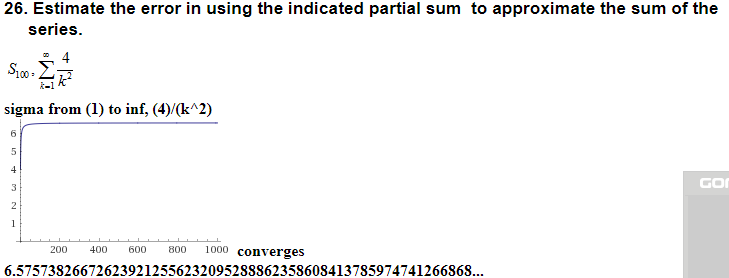# 26. Estimate the error in using the indicated partial sum to approximate the sum of theseries.sigma from (1) to inf, (4)/(k*2)GO200 400 60 800 1000 converges6.575738266726239212556232095288862358608413785974741266868...

Question

### 26. Estimate the error in using the indicated partial sum  to approximate the sum of the series.

sigma from (1) to inf, (4)/(k^2)

converges

6.575738266726239212556232095288862358608413785974741266868..help_outlineImage Transcriptionclose26. Estimate the error in using the indicated partial sum to approximate the sum of the series. sigma from (1) to inf, (4)/(k*2) GO 200 400 60 800 1000 converges 6.575738266726239212556232095288862358608413785974741266868... fullscreen
check_circleExpert Solution
Step 1

It is given that the sum of series is

Step 2

The partial sum of the series can be computed as

Step 3

By the remainder estimate for th...

### Want to see the full answer?

See Solution

#### Want to see this answer and more?

Solutions are written by subject experts who are available 24/7. Questions are typically answered within 1 hour*

See Solution
*Response times may vary by subject and question
Tagged in

### Calculus